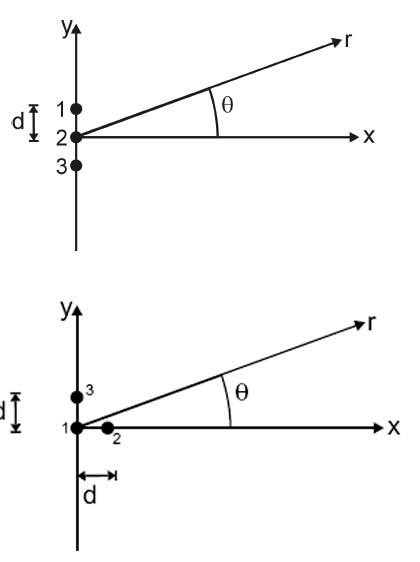# Superposition of waves

leopardFigure on top:
$$P_{1}= (1/16)W, P_{2}=1W, P_{3}= 16W$$, and I want to calculate how the intensity varies with $$\theta$$

$$y(r,t) = y_{2}(r,t)[1 + 4e^{i(\phi_{3} - \phi_{2} + kdsin \theta} + \frac{1}{4} e^{i(\phi_{1} - \phi_{2} - kd sin \theta)}]$$

I understand how to proceed here, I just want to know how to determine whether it's + or - in front of $$\phi$$s and $$kdsin \theta$$

Bottom figure:

$$P_{1}=P_{2}=P_{3} = 10W$$, same problem.

$$y(r,t)=y_{1}(r,t)[1 + e^{i(\phi_{2} - \phi_{1} + kd cos \theta)} + e^{i(\phi_{3} - \phi_{1} + kd sin \theta)}]$$

Is it just me, or is there an incoherence here, as how to determine the signs? Both are from exam solution manuals at my university, so it should be correct.